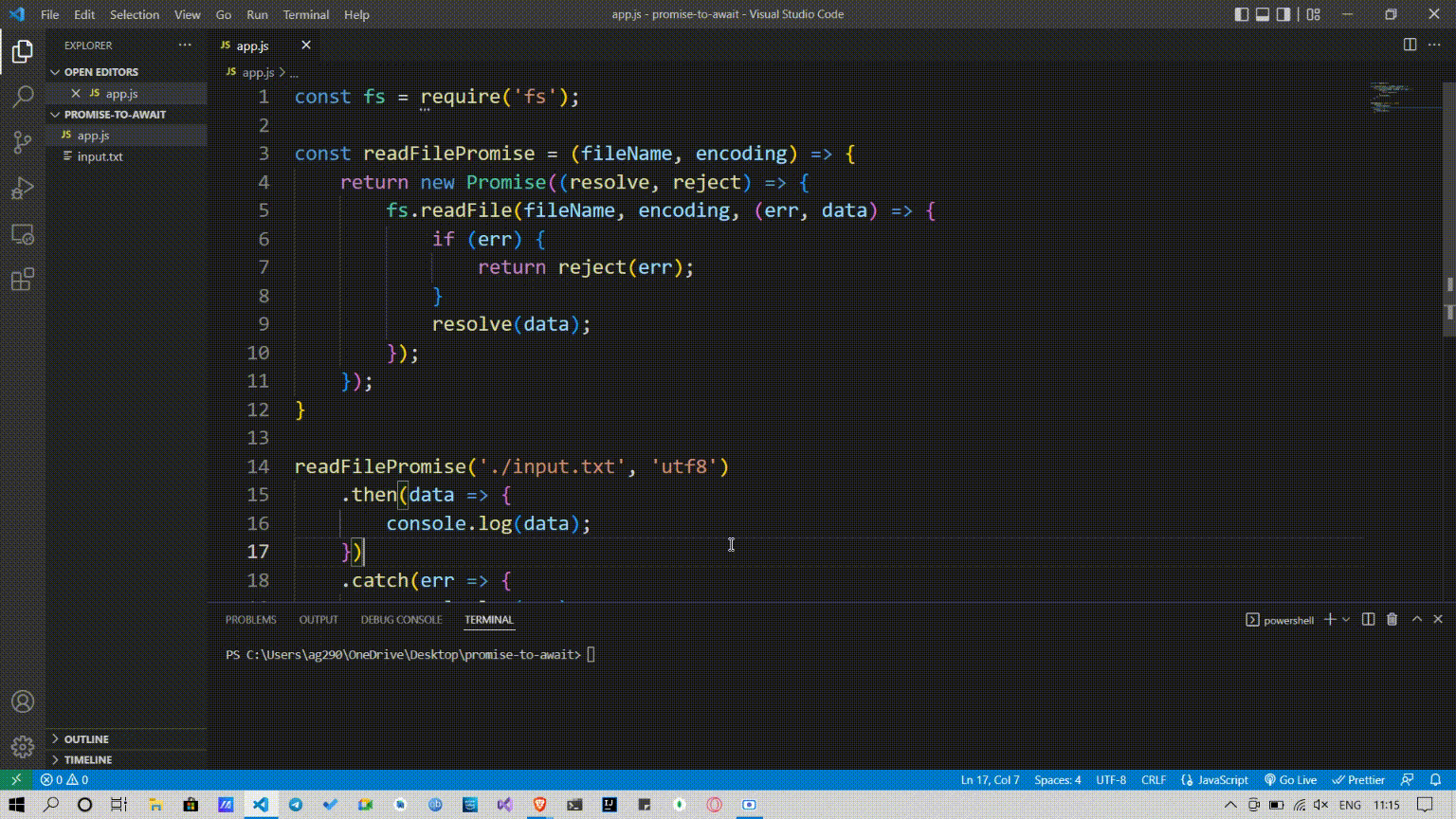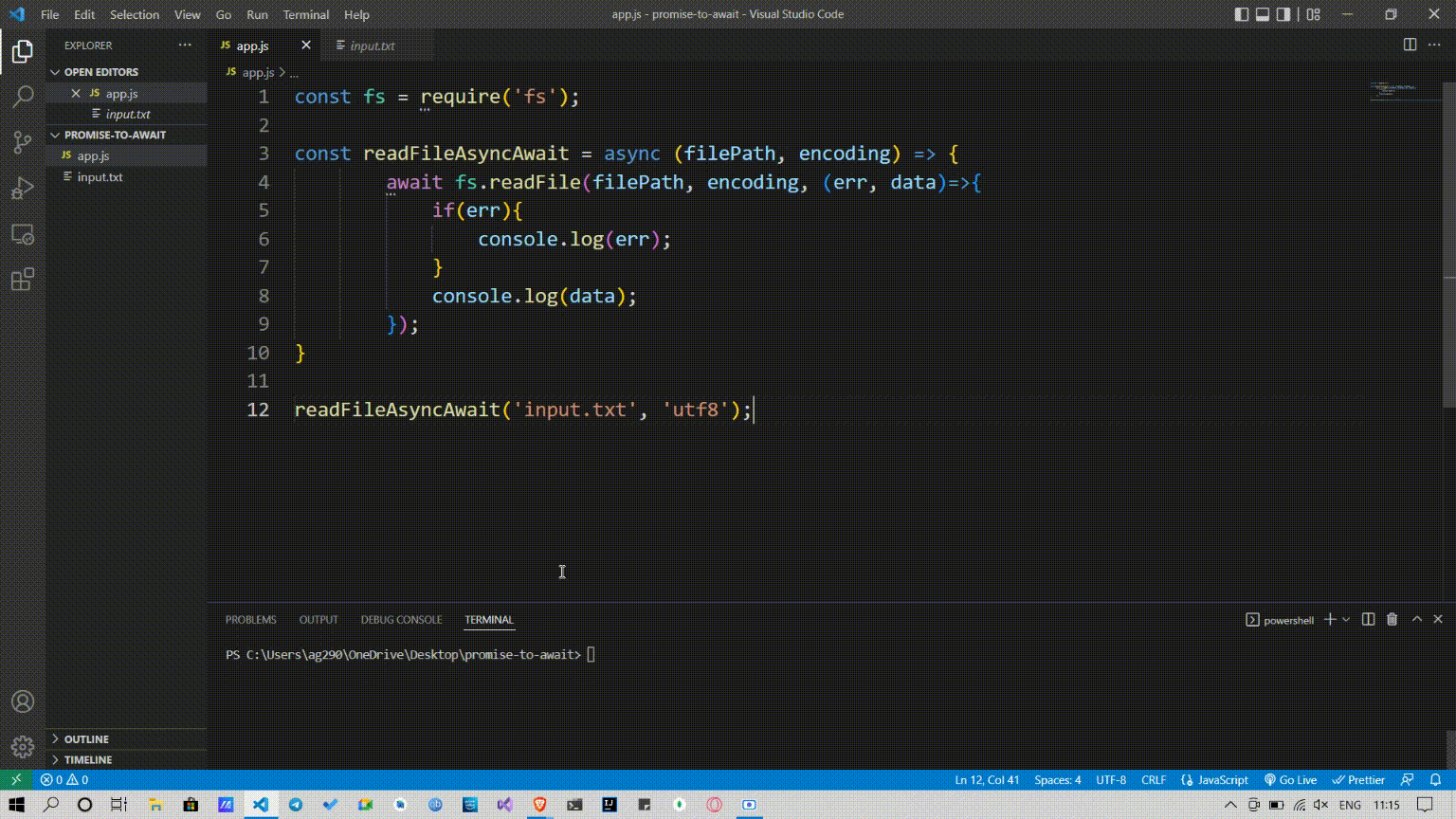# How to Rewrite promise-based applications to Async/Await in Node.js ?

• Last Updated : 19 May, 2022

In this article, we are going to see how we can rewrite the promise-based Node.js application to Async/Await.

Suppose we have to read the content of a file ‘index.txt’, and we have a function ‘readFilePromise’ which uses the ‘readFile’ method of the fs module to read the data of a file, and the’readFilePromise’ function resolves the promise if the data is found otherwise rejects the promise with respective error.

```const fs = require('fs');

const readFilePromise = (fileName, encoding) => {
return new Promise((resolve, reject) => {
fs.readFile(fileName, encoding, (err, data) => {
if (err) {
return reject(err);
}
resolve(data);
});
});
}```

then, we can handle data by calling the ‘readFilePromise’ function with two arguments, the file path, and the file encoding, if it gets the required output then it executes the code of then block, and if it gets the error then it executes the code of the catch block.

```readFilePromise('./input.txt', 'utf8')
.then(data => {
console.log(data);
})
.catch(err => {
console.log(err);
});```

Example:

## Javascript

 `const fs = require(``'fs'``);`` ` `const readFilePromise = (fileName, encoding) => {``    ``return` `new` `Promise((resolve, reject) => {``        ``fs.readFile(fileName, encoding, (err, data) => {``            ``if` `(err) {``                ``return` `reject(err);``            ``}``            ``resolve(data);``        ``});``    ``});``}`` ` `readFilePromise(``'./input.txt'``, ``'utf8'``)``    ``.then(data => {``        ``console.log(data);``    ``})``    ``.``catch``(err => {``        ``console.log(err);``    ``});`

Output:Now let’s rewrite this promise to async/await. For using ‘await’, we have to use the ‘async’ keyword, ‘await’ to hold the execution of the program till the ‘readFile’ method of the fs module returns something. It can be an error or required data, if it is an error then we have to log the error, if not then log the required data.

```const fs = require('fs');

const readFileAsyncAwait = async (filePath, encoding) => {
if(err){
console.log(err);
}
console.log(data);
});
}```

Then we can call the ‘readFilePromise’ function with two arguments, the file path, and the file encoding, and if there is any error, it simply logs the error otherwise log the required data.

`readFileAsyncAwait('input.txt', 'utf8');`

Example:

## Javascript

 `const fs = require(``'fs'``);`` ` `const readFileAsyncAwait = async (filePath, encoding) => {``    ``await fs.readFile(filePath, encoding, (err, data)=>{``        ``if``(err){``            ``console.log(err);``        ``}``        ``console.log(data);``    ``});``}`` ` `readFileAsyncAwait(``'input.txt'``, ``'utf8'``);`

Output:My Personal Notes arrow_drop_up